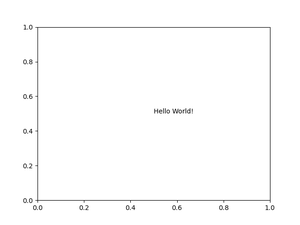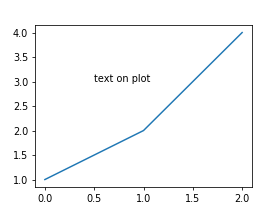# Matplotlib.pyplot.text() function in Python

• Difficulty Level : Easy
• Last Updated : 25 Nov, 2020

This function is used to add a text to the axes at location x, y in data coordinates.

`Syntax: matplotlib.pyplot.text(x, y, s, fontdict=None, **kwargs)`

Example #1: Text on plot sheet

Attention geek! Strengthen your foundations with the Python Programming Foundation Course and learn the basics.

To begin with, your interview preparations Enhance your Data Structures concepts with the Python DS Course. And to begin with your Machine Learning Journey, join the Machine Learning - Basic Level Course

## Python3

 `import` `matplotlib.pyplot`` ` `matplotlib.pyplot.text(``0.5``, ``0.5``, ``"Hello World!"``)``matplotlib.pyplot.savefig(``"out.png"``)`

Output:Example #2: Add text to a plot

## Python3

 `import` `matplotlib.pyplot as plt`` ` ` ` `w ``=` `4``h ``=` `3``d ``=` `70`` ` `plt.figure(figsize``=``(w, h), dpi``=``d)`` ` `x ``=` `[``1``, ``2``, ``4``]``x_pos ``=` `0.5``y_pos ``=` `3`` ` `plt.text(x_pos, y_pos, ``"text on plot"``)``plt.plot(x)``plt.savefig(``"out.png"``)`

Output:My Personal Notes arrow_drop_up